23.02.2021 — Hasnain Raza

# Opening the black box of vision AI algorithms

A pattern-matching view on convolutions and neural networks

Deep learning is everywhere nowadays, from your smartphone camera to your smart speaker. However, many people think that deep learning algorithms “think” and make conscious decisions because, after all, they are modeled after the brain. However, the reality is far from it. In the end, a neural network is nothing more than a mathematical function; a VERY VERY VERY complicated function, but a clearly defined function with predictable outputs. In short: “AI” is not intelligent yet, but can produce results that have some semblance of intelligence.

To illustrate this “dumb AI” paradigm, we will explain the intuition behind convolutional neural networks (CNNs) — the foundation for most SOTA (state of the art) models in computer vision. We’ll show you how deep learning on images actually works down to its most basic idea while staying away from fancy jargon and complex math. Our goal is to open the black box of how vision AI works for people who don’t have a background in machine learning. But also for proven ML-practitioners, it doesn’t hurt to reinforce the intuition behind CNNs. By understanding how convolutions work, you’ll be aware of vision AI’s limitations and understand that this is not a one-size-fits-all, magic bullet solution to every problem.

We’ll provide some simple code examples along the way so you can play around with the code and get an even more in-depth understanding of the topic.

## A quick recap: convolutional neural networks

Let’s start with some theory. As explained above, a neural network is a mathematical function. A simple example of a function is y = 2a; this function accepts a number (a) and outputs another number (y). It also doubles whatever the input is.
For vision AI applications, the input of the function is an image. The output varies depending on the application, but usually, it’s some sort of a prediction in the form of probabilities. Let’s say you want to build a model to classify dogs and cats. Here the output will be a set of probabilities, as shown:

(PDog, PCat) = (x%, y%)

This means that the model predicts with a confidence of x% that it’s an image of a dog, and with a confidence of y%, it’s an image of a cat. Notice one interesting thing here; there is no way for the neural network to answer: “I don’t know”.

Each neural net is built of a set of sub-functions, also called layers. The input (image) is passed to the first layer, making calculations, and then gives the output to the next layer, using it as input. This process is repeated until the final output layer is reached.

The core building block of a CNN is a function called convolution. This blogpost (as mentioned above) aims at developing an intuition for how convolutions work so that we may understand how CNNs operate as functions/number crunchers. And not conscious decision-makers. Figure 1 shows the composition of a simple CNN.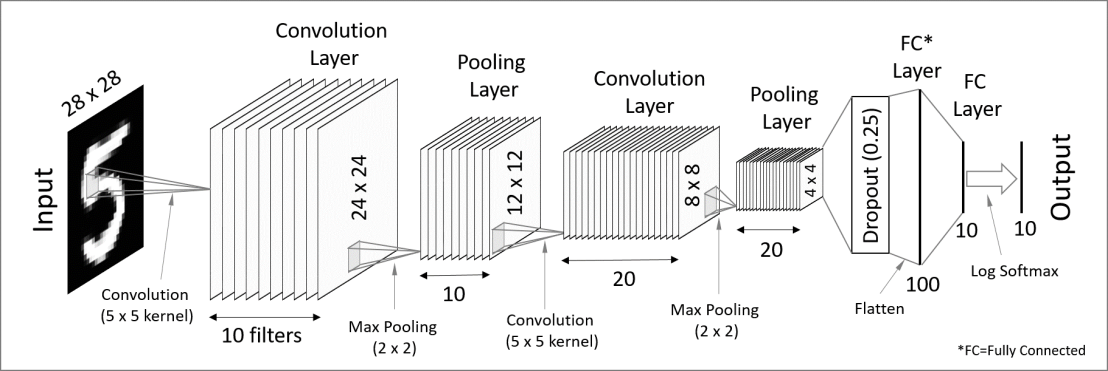Figure 1: A simple CNN. The diagram shows just how much of it is comprised of convolutional layers. The other layers (like pooling and dropout) are there as add-ons and not necessities to the model itself. Source

## Understanding convolutions with pattern matching

The aim here is to introduce convolutions by looking at pattern matching first, which is a bit simpler. We’ll be following a simple toy problem (to make the math and thought process linear and easy).

Let’s create an image to work with during our example here. For this, we need to understand how a computer stores images:

Looking at grayscale images as an example, the image is a grid of numbers. Each element in the grid (pixel) represents the intensity of white. 0 is black, 255 is white. The same logic can be extended to color images. Each pixel is represented by 3 numbers, each representing the intensity of red, green, and blue. By interpreting each RGB value, a computer can display color images.

Note here for a reminder: a “grid of values” we speak of is what’s known as a matrix in mathematics or an array in computer science. They all refer to the same thing.

For our dummy-image here, we’ll be using binary images (only two colors, black and yellow, 0 is black, 1 is yellow) to illustrate convolutions pictorially. To create such an image in python, we’ll need to import some libraries first:

``````{
import numpy as np
import matplotlib.pyplot as plt
plt.rcParams['image.cmap'] = 'magma'
}``````

Let’s create the 3x3 binary image with a horizontal line in the middle:

``````{
sample_image = np.zeros(shape=(3, 3))
sample_image[:, 1] = 1
plt.imshow(sample_image)
plt.axis('off')
}``````

Output: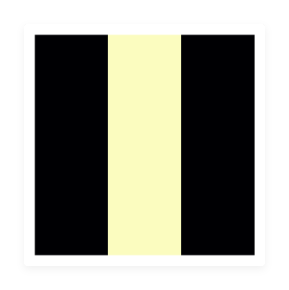Figure 2: Binary sample image. Yellow is 1, and Black is 0. A 3-pixel long line in the middle.

Alright, now let’s pose the first question to help us to get us moving: Can you write an algorithm that will find a 3-pixel long straight line in the middle of any given binary image?

One idea, amongst many that you may come up with, is template matching. We know what we are looking for, so why not just create a template out of it? That sounds easy enough; let’s do it:

``````{
template = sample_image.copy()
plt.imshow(template)
plt.axis('off')
}``````

Output:Figure 3: Template image — The pattern we will be looking for (3-pixel long straight line in the middle)

As expected, the template looks like what we are looking for (a 3-pixel long line in the center). It is also identical to our image for this case.

We have the template, now we need the matching. For the case above, how can we ensure a match?

Here comes the most difficult part of this post: elementary math. If we do element-by-element multiplication of the two binary matrices (image and template) and sum up all the resulting elements, it should equal 3 if it is a match.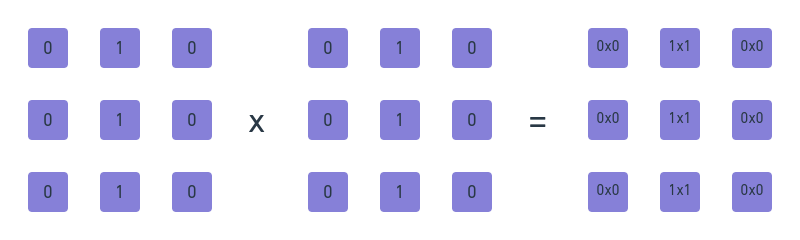Figure 4: Element-wise multiplication of two matrices, notice how the result has 3 1s and 6 0s, summing all these numbers up would equal 3.

Now, let’s implement this simple algorithm:

``````{
result_multiplication = sample_image * template
value = np.sum(result_multiplication)
if value == 3:
print("It's a match!")
else:
print("Not a match")
}``````

Our algorithm above outputs “it’s a match” meaning that our image and template are an exact match.

Now, let’s make things a bit more interesting and not compare identical images and templates. The code below simply runs our template matching algorithm on random 3x3 binary images.

``````{
def template_matcher(image, template, value, i):
fig, ax = plt.subplots(1, 2)
ax.imshow(image, cmap='magma')
ax.axis('off')
ax.imshow(template, cmap='magma')
ax.axis('off')
plt.savefig(f'{i}.png', bbox_inches='tight')
plt.show()
output = np.sum(image * template)
if output == value:
print("Match")
else:
print("Not a Match")

for i in range(5):
image = np.random.randint(0, 2, size=(3, 3))
template_matcher(image, template, 3, i)
}``````

Output: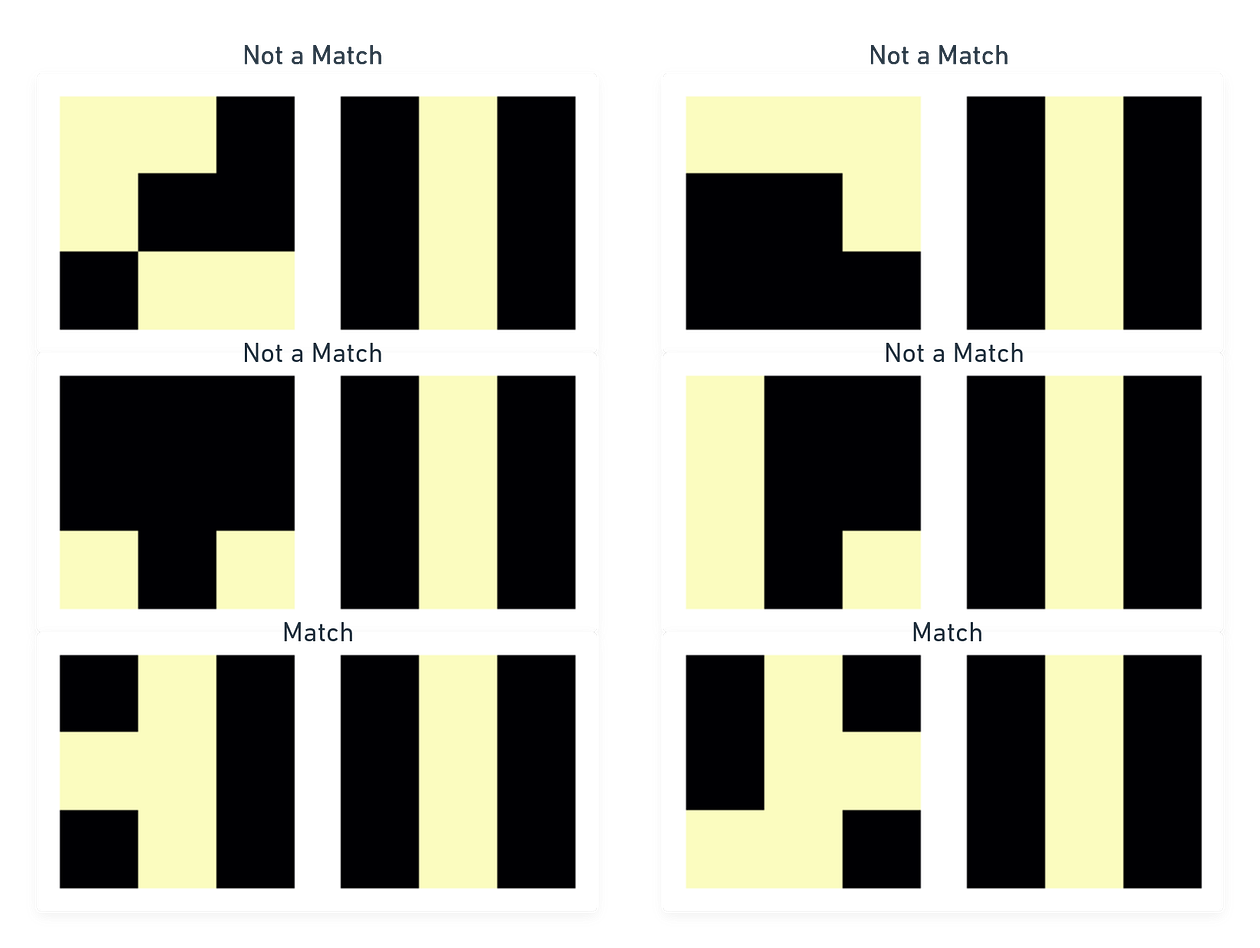Figure 5: Image-Template pairs output, first two rows show no match, the last row shows examples where a template is a match.

## Why are we doing multiplications but talking about convolutions?

Above, we showed you how to match patterns in a 3x3 image, but this is not useful for images coming from your phone camera. One part of this being that they are gigantic compared to our small 3x3 toy image. So now, when your image is 3000x3000 pixels, how would you do element by element multiplication? There aren’t enough elements in our small 3x3 template. You might think that one solution could be to increase the template size as well. But you will have to realize that to find 3-pixel lines in different areas of the image, you’ll need more and more templates, as shown in Figure 5: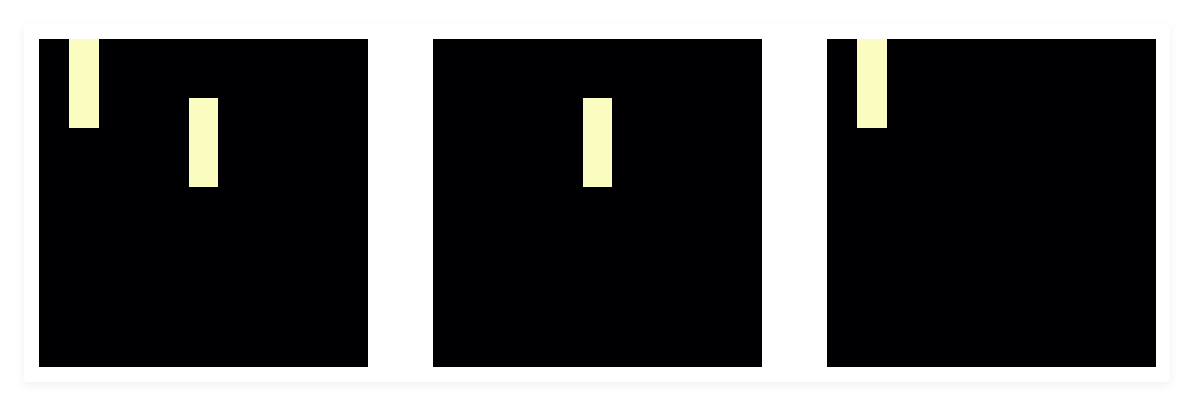Figure 6: Left most is an 11x11 image. The Middle and Right images depict the templates required to find each of the lines if we make our templates bigger to match the image size.

According to figure 6, to cover all possible regions in an 11x11 image where a 3-pixel long line would be, we would need 11 x 9 = 99 (11 columns, 9 positions in each column for a 3-pixel long line) templates. See how quickly it blew up for such a simple problem? We not only need more memory for each of these templates, but we also need more multiplications now.

Luckily, there’s a smarter way to go about this. We can keep the template small (3x3) but use a sliding window for the template and still do the element-by-element multiplication we’ve been doing between image and template. Do it on smaller chunks of the image, such that the chunk and the template have the same size.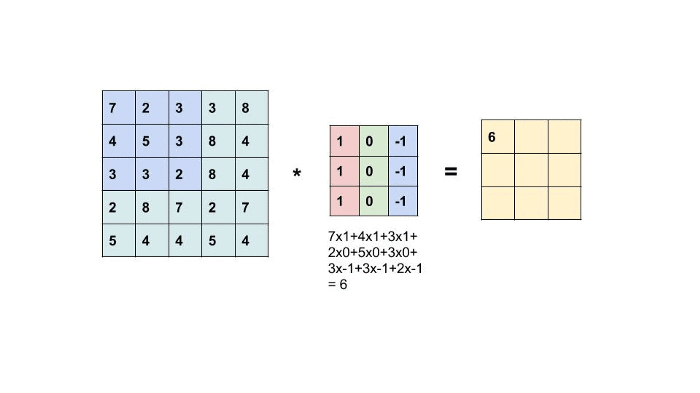Figure 7: Convolution operation. Bigger image (left), smaller template (Middle), output result (Right). The gif is taken from this Medium Post.

As a result, we get a map of where we find our pattern in the image (every field containing a three) without creating any additional templates.

``````{
def template_matcher(image, template, value, i):
fig, ax = plt.subplots(1, 2)
ax.imshow(image, cmap='magma')
ax.axis('off')
ax.imshow(template, cmap='magma')
ax.axis('off')
plt.savefig(f'{i}.png', bbox_inches='tight')
plt.show()
output = np.sum(image * template)
if output == value:
print("Match")
else:
print("Not a Match")

for i in range(5):
image = np.random.randint(0, 2, size=(3, 3))
template_matcher(image, template, 3, i)
}``````

Our image and our template:Figure 8: Left is the 11x11 image generated by the code block above, right is the 3x3 template we will convolve the image with.

The output:Figure 9: Output of our convolution. Yellow represents a value of 3, peach represents 2, purple represents 1. As can be seen, values of 2 and 1 were produced as well since the template was only able to match 2 pixels and 1 pixel as it moved down during convolution on the left side of the image.

If you are curious about the specifics, we recommend going through the excellent course notes of CS231n by Stanford University on optimization and learning.

Let’s assume we don’t know what the template is. But we do have data, lots and lots of labeled data. We also will be using element-by-element multiplication, but keep in mind that convolutions are simply the same operation on smaller chunks of the image. Intuitively, both are equal. Code below shows our labeled data producer (comes from some oracle, in the real world, this labeling is done by human beings, here, we can simply code this oracle knowledge since the problem is so easy):

``````#This is the learned template, the one we want to learn, we initialize it randomly
# This is the optimizer, it does all the calculus and derivatives for us
optimizer = torch.optim.SGD([learned_template], lr=0.1)``````
``````# We train for a couple of iterations
for train_iteration in range(1000):
# Clean out any old gradients
# Get the image and ground truth
image, label = generate_image_and_ground_truth_label()
# Calculate output, scale it by 3 (max value is 3, this is optional)
y = torch.sum(learned_template * image) / 3
# Calculate loss
loss = (y - label) ** 2
# Backprop loss (calculate the influence of learned_template on the loss)
loss.backward()
# Perform update (Change learned_paramter given the influences)
optimizer.step()``````

Above, we chose to model this problem the same way we’ve been doing throughout this post (multiply image and template together, sum results). This allows us to check the error against the label the human being has created. If the error (loss in the code block) is high, that means our template is not correct, and the loss is used to calculate how to tune the values in the template such that its prediction gets better. We do this repeatedly, about 1000 times until finally, we get the learned template. Notice how it resembles the oracle template (high values in the middle column, low values in the extreme columns). The minor differences in colors and pixel values are because the learned template consists of real numbers while our oracle is made up of binary numbers.Figure 10: Learned Template (left), Oracle Template (right)Figure 11: Visualisation of actually trained convolution layers of a network to recognize all sorts of objects. From the first convolutional layer of AlexNet. Source

## Conclusion

As can be seen, the training process simply tunes numbers in the templates (or kernels or filters as they are called) to make the convolutional neural network decisions correct. At the end of the day, it is a bunch of matrices being multiplied with the input. Changing the input values to be different from what they were when the network was “trained” will result in the neural network making unexpected predictions simply because the templates were not found for this new data. Similarly, since many matrices are being multiplied together, there is no conscious decision-making at play there. Though there is a deeper philosophical argument if we go down the road of asking questions like: What is intelligence? Can a big enough neural network give rise to consciousness through the phenomenon of emergence?

We hope that we could open the black box of vision AI algorithms and provide some intuition of how an AI is not making conscious decisions but is just multiplying a LOT of matrices.

## Shameless plug time

Only 13% of vision AI projects make it to production. With Hasty, we boost that number to 100%.
Our comprehensive vision AI platform is the only one you need to go from raw data to a production-ready model. We can help you with:

All the data and models you create always belong to you and can be exported and used outside of Hasty at any given time entirely for free.

You can try Hasty by signing up for free here. If you are looking for additional services like help with ML engineering, we also offer that. Check out our service offerings here to learn more about how we can help.